Multi-Dimensional Arrays in C Programming

Consider a 3X3 matrix. It has 3 rows and 3 columns. When we say 3 rows and 3 columns, it means that each row has 3 elements or each column has 3 rows. This can be stated in C language as each row is an array of 3 elements or each column is an array of 3 elements. When we combine all the rows or columns of this matrix, it becomes array of arrays. That means arrays Rows (Arrays of columns).

We can use this same concept to represent the matrix using C language. That means, elements in rows and columns are considered as arrays of arrays and they can be used to store the values. This arrays of array is called as 2 dimensional array. This same concept when extended to store more dimensional values, it is called as multidimensional array.

Let us consider 2D array now. As we discussed above 2D array is an array of rows with array of columns. Consider 3X3 matrix below. It has 3 rows and 3 columns. Each row is an array of 3 elements.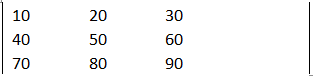This can be considered as an 2D array and can be represented in C as below.

int intArr  ;

Here intArr is a 2D array with 3 rows (first [] element) and 3 columns (second [] element) of integer types. That means its elements are of integer types. Though we say it as 2D array, in memory they occupy contiguous memory locations, without any separation between rows / columns, like one dimensional array. This can be differentiated while coding by the user. Each element in the 2D array are accessed by using the indexes like 1D array – as a convenient method first index always represents row and second index represents the column. i.e.; intArr  represent the first row, 3rd column element of the array, i.e.; 30.

Storage Classes in C Programming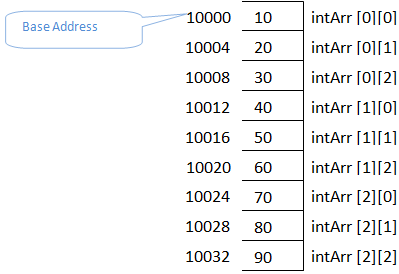A 2D array can be initialized in the same way as we did for 1D array. Here we need not differentiate rows and columns. It will automatically assign the row and column values depending upon the dimensions specified while declaring the array.

While declaring the array variable

This works similar to 1D array. Any uninitialized array elements will have 0 assigned to it in this method.

int intArr  = {10, 20, 30, 40, 50, 60, 70, 80, 90, 100}; // initializes all the 10 elements
int intArr  = {10}; // initializes first element to 10 and rest of them will be zero

#include <stdio.h>

void main () {
int intArr  = { 5, 10, 15, 20, 25, 30, 35, 40, 45 };

printf ("\nElements of array are:\n");
for (int i = 0; i < 3; i++){
for (int j = 0; j < 3; j++)
printf ("%d\t", intArr[i] [j]);
printf ("\n"); // helps display in table format
}
}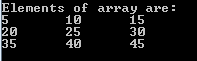Here the elements of 2D arrays are initialized without considering rows and columns. But the dimensions mentioned while declaring intArr decides the number of rows and columns and divides the elements into their respective rows and columns. It is displayed in the form of table because of the printf statement in the outer most for loop. If we remove that statement, we will not be able to differentiate any rows or columns and will see the 2D array data as 1D array elements.

Here also, we need not specify the dimension of the array while declaring the array, if we are initializing too. But it is required to mention the number of column in array declaration, so that number of rows can be automatically determined. Otherwise it will be same as single dimensional array.

#include <stdio.h>

void main () {
int intArr [] = { 5, 10, 15, 20, 25, 30, 35};

printf ("\nElements of array are:\n");
for (int i = 0; i < 3; i++){
for (int j = 0; j < 3; j++)
printf ("%d\t", intArr[i] [j]);
printf ("\n"); // helps display in table format
}
}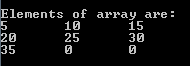In the example above, we have not mentioned the number of rows. But we have specified the number of columns. Hence when we initialize the elements of it, after each 3rd element, it considers the next element as the element of next row. Hence it automatically determined that it has 3 rows. In addition, we have not initialized all the elements of the array. It has automatically initialized them to zero.

Variables in C Programming

After declaring array variable

If we are initializing the array after declaring them, then we need to individually select the elements of the array to assign the values to them, like we did in 1D array.

int intArr ;

intArr  = 10;
intArr [1} = 20;
intArr  = 30;

#include <stdio.h>

void main(){
int intArr ;

intArr  = 10;
intArr = 20;
intArr  = 30;

printf ("\nElements of array are:\n");
for (int i = 0; i < 3; i++) {
for (int j = 0; j < 3; j++)
printf ("%d\t", intArr[i][j]);
printf ("\n");
}
}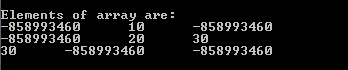Here we can notice that, unlike first method of initializing, any uninitialized elements of the array will have garbage values. Only those elements that are initialized have the correct values. We can notice one more 30 at 2nd row 3rd column which is not initialized value; but it is some garbage value (garbage value can be any value which user has not entered).

By entering the values from keyboard or input file

User can be asked to input the values to the array by using scanf function. This will assign values to the each elements in the array like below.

#include <stdio.h>

void main (){
int intArr ;

printf ("\nPlease integer numbers into two dimensional array :");
for (int i = 0; i < 3; i++)
for (int j= 0;j < 3;j++)
scanf ("%4d", &intArr[i][j]);

printf ("\nElements of array are:\n");
for (int i = 0; i < 3; i++){
for (int j = 0; j < 3; j++)
printf ("%d\t", intArr[i][j]);
printf ("\n");
}
}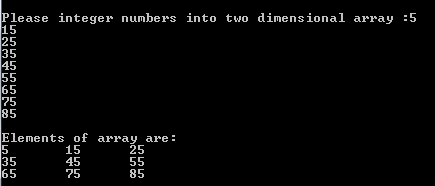This is how a 2D array is declared, initialized and accessed. We can even have more than 2 dimensional arrays which are termed as multidimensional array. It can be declared initialized in the same way as 2D array. Examples of declaring, initializing and accessing multidimensional array are as below: